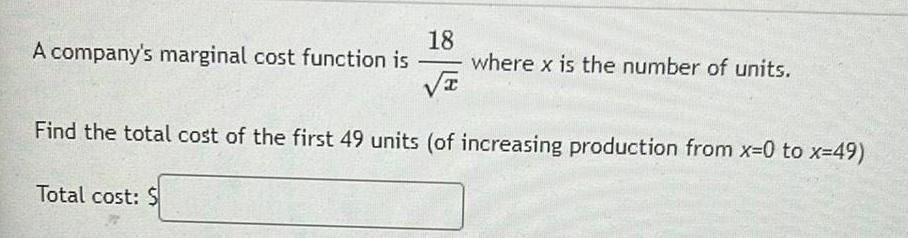Question:

# 18 A company's marginal cost function is where x is the

Last updated: 8/12/202218 A company's marginal cost function is where x is the number of units. Find the total cost of the first 49 units (of increasing production from x=0 to x=49) Total cost: S